Courses

Subjective Type Questions: Electrostatics- 2 | JEE Advanced Notes | Study Physics 35 Years JEE Main & Advanced Past year Papers - JEE

JEE: Subjective Type Questions: Electrostatics- 2 | JEE Advanced Notes | Study Physics 35 Years JEE Main & Advanced Past year Papers - JEE

The document Subjective Type Questions: Electrostatics- 2 | JEE Advanced Notes | Study Physics 35 Years JEE Main & Advanced Past year Papers - JEE is a part of the JEE Course Physics 35 Years JEE Main & Advanced Past year Papers.
All you need of JEE at this link: JEE

Q.13. A circular ring of radius R with uniform positive charge density λ per unit length is located in the y-z plane with its centre at the origin O. A particle of mass m and positive charge q is projected from the point P (R√ 3,0,0) on the positive x-axis directly towards O, with an initial speed v.

Find the smallest (non-zero) value of the speed v such that the particle does not return to P.       (1993-4 Marks)

Ans.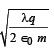Solution. Potential energy can be found at the initial point A and final point O. The difference in potential energy has to be provided by the K.E. of the charge at A.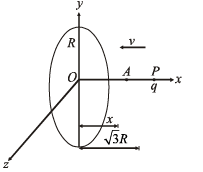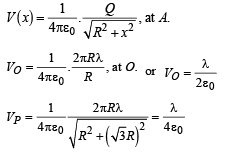Potential difference between points O and P = V

∴ V = VO-VP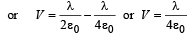The kinetic energy of the charged particle is converted into its potential energy at O.

∴ Potential energy of charge (q) = qV

Kinetic energy of charged particle = 1/2 mv2

For minimum speed of particle so that it does not return to P,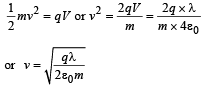Q.14. Two square metal plates of side 1 m are kept 0.01 m apart like a parallel plate capacitor in air in such a way that one of their edges is perpendicular to an oil surface in a tank filled with an  insulating oil. The plates are connected to a battery of emf 500 V. The plates are then lowered vertically into  the oil at a speed of 0.001 ms–1. Calculate the current drawn from the battery during the process. (Dielectric constant of oil = 11, ε0 = 8.85 × 10–12C2N–1m–1)       (1994 - 6 Marks)

Ans. 4.425 × 10–9 A.

Solution. The adjacent figure is a case of parallel plate capacitor. The combined capacitance will be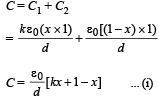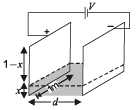Differentiating the above quation w.r.t. time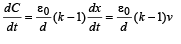where v = dx/dt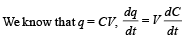From (iii) and (iv)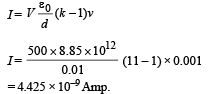Q.15. The capacitance of a parallel plate capacitor with plate area A and separation d is C. The space between the plates is filled with two wedges of dielectric constants K1 and K2, respectively. Find the capacitance of the resulting capacitor.       (1996 - 2 Marks)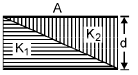Ans.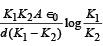Solution. Case (i) When no dielectric :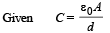Case (ii) When dielectric is filled : A small dotted element of thickness dx is considered as shown in the figure.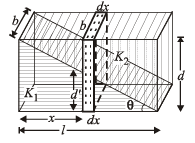The small capacitance of the dotted portion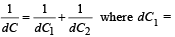capacitance of capacitor with dielectric K1

dC2 = capacitance of capacitor with dielectric K2.

Let ℓ, b the len gth an d br eadth of the capacitor plate.

Therefore ℓ × b=A.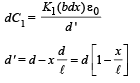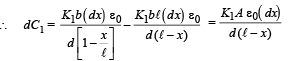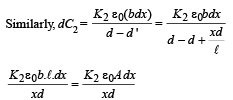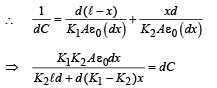To find the capacitance of the whole capacitor, we integrate the above equation.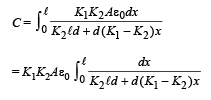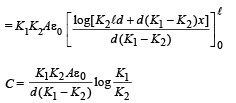Q.16. Two capacitors A and B with capacities 3 μF and 2 μF are charged to a potential difference of 100 V and 180 V respectively. The plates of the capacitors are connected as shown in the figure with one wire from each capacitor free.

The upper plate of A is positive and that of B is negative. An uncharged 2 μF capacitor C with lead wires falls on the free ends to complete the circuit. Calculate         (1997 - 5 Marks)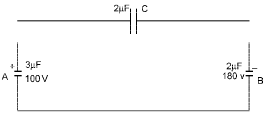(i) the final charge on the three capacitors. and
(ii) the amount of electrostatic energy stored in the system before and after the completion of the circuit.

Ans. (i) 90 × 10–6 C, 210 × 10–6 C, 150 × 10–6 C
(ii) 4.74 × 10–2 J, 1.8 × 10–2 J

Solution. (i) KEY CONCEPT : Use charge conservation to solve this problem.

INITIALLY :

Charge on capacitor A

qA = 3  × 10–6 × 100 = 3 × 10–4C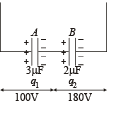Charge on capacitor B

qB = 2  × 10–6 × 180 = 3.6 × 10–4C

FINALLY :

Let the charge on capacitor A, C and B be q1, q2 and q3 respectively.

By charge conservation.

The sum of charge on +ve plate of capacitor A and C should be equal to qA

∴ q1 + q2 = 3 × 10–4C... (i)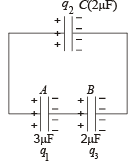Similarly the sum of charge on –ve plates of capacitor C and B will be equal to qB

∴ – q2 – q3 = – 3.6 × 10–4

⇒ q2 + q3 = 3.6 × 10–4C ... (ii)

Applying  Kirchoff's law in the closed loop, we get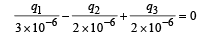⇒ 2q1 – 3q2 + 3q3 = 0 ....(iii)

On solving (i), (ii) and (iii), we get

q1 = 90 × 10–6 C, q2 = 210 × 10–6 C, and q3 = 150 × 10–6 C,

(ii) Amount of electrostatic energy in the system initially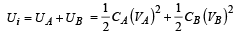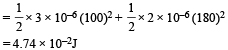Amount of electrostatic energy stored finally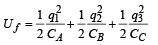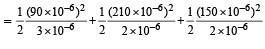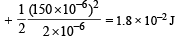Q.17. A conducting sphere S1 of radius r is attached to an insulating handle. Another conducting sphere S2 of radius R is mounted on an insulating stand. S2 is initially uncharged.

S1 is given a charge Q, brought into contact with S2, and removed. S1 is recharged such that the charge on it is again Q; and it is again brought into contact with S2 and removed.

This procedure is repeated n times.             (1998 - 8 Marks)

(a) Find the electrostatic energy of S2 after n such contacts with S1.
(b) What is the limiting value of this energy as n →∞ ?

Ans.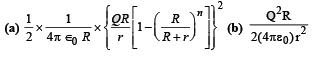Solution.  Limiting value of energy as n →∞.

Let us calculate qn when n tends to ∞.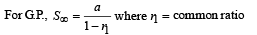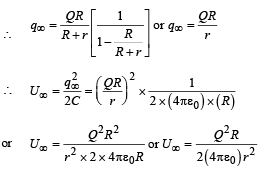Q.18. A non-conducting disc of radius a and uniform positive surface charge density σ is placed on the ground, with its axis vertical. A particle of mass m and  positive charge q is dropped, along the axis of  the disc, from a height H with zero initial velocity. The particle has q/m = 4 ∈0g/σ    (1999 - 10 Marks)

(a) Find the value of H if the particle just reaches the disc.
(b) Sketch the potential energy of the particle as a function of its height and find its equilibrium position

Ans.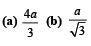Solution. (a) KEY CONCEPT :  The K.E. of the particle, when it reaches the disc is zero.

Given that a = radius of disc, σ = surface charge density, q/m = 4ε0g/σ

Potential due to a charged disc at any axial point situated at a distance x from O is,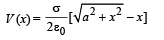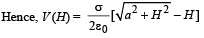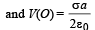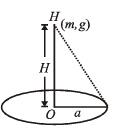NOTE : According to law of conservation of energy, loss of gravitational potential energy = gain in electric potential energy

mgH = qΔV

= q [V(0) – V (H)]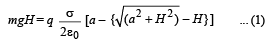From the given relation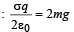Putting this in equation (1), we get,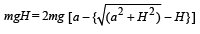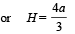[∵H = O is not valid]

(b) Total potential energy of the particle at height H

U (x) = mgx + qV (x)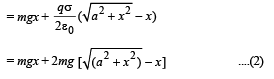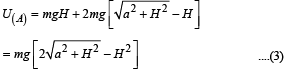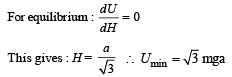From equation (2), graph between U(x) and x is as shown above.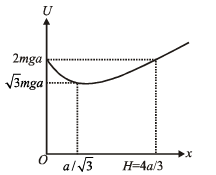Q.19. Four point charges +8mC, –1mC, –1mC, and +8mC are fixed at the points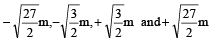respectively on the y-axis. A particle of mass 6 × 10–4 kg and charge +0.1 μC moves along the -x direction. Its speed at x = + ∞ is V0. Find the least value of V0 for which the particle will cross the origin. Find also the kinetic energy of the particle at the origin. Assume that space is gravity free.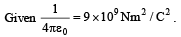(2000 - 10  Marks)

Ans. 3 m/s,  3 × 10–4 J

Solution. Let the particle at some instant be at a point P distant x from the origin. As shown in the figure, there are two forces of repulsion acting due to two charges of + 8 mC. The net force is 2F cos α towards right.

Similarly there are two forces of attraction due to two charges of – 1 mC. The net force due to these force is 2F' cos β towards left.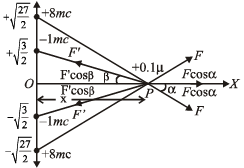The net force on charge 0.1 µC is zero when 2F cos α = 2F' cos β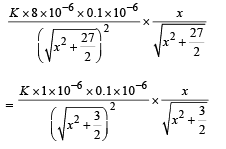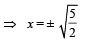This means that we need to move the charge from – ∞ to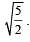Thereafter the attractive forces will make the charge move to origin.

The electric potential of the four charges at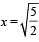is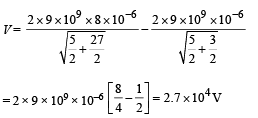Kinetic energy is required to overcome the force of repulsion

from ∞ to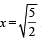The work done in this process is W = q (V)

where V = p.d between ∞ and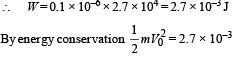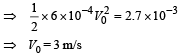K.E. at the origin

Potential at origin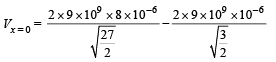= 2.4 × 104

Again by energy conservation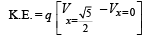∴K.E. = 0.1 × 10–6 [2.7 × 104 – 2.4 × 104]

= 0.1 × 10–6 × 0.3 × 104

= 3 × 10–4 J

Q.20. Charges +q and –q are located at the corners of a cube of side as show in the figure. Find the work done to separate the charges to infinite distance.          (2003 - 2 Marks)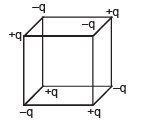Ans.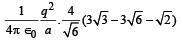Solution.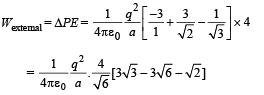Q.21. A charge +Q is fixed at the origin of the co-ordinate system while a small electric dipole of dipole moment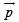pointing away from the charge along the x-axis is set free from a point far away from the origin.       (2003 - 4 Marks)

(a) Calculate the K.E. of the dipole when it reaches to a point (d, 0).

(b) Calculate the force on the charge +Q at this moment.

Ans.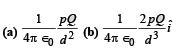Solution. (a) Potential energy of the dipole-charge system

Ui = 0 (since the charge is far away)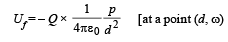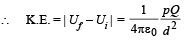(b) Electric field at origin due to dipole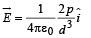Thus, force on charge Q is given by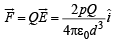Q.22. Two uniformly charged large plane sheets S1 and S2 having charge densities σ1 and σ21 > σ2) are placed at a distance d parallel to each other. A charge q0 is moved along a line of length a(a < d) at an angle 45º with the normal to S1. Calculate the work done by the electr ic field         (2004)

Ans.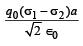Solution.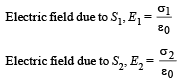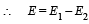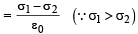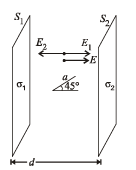Work done by electric field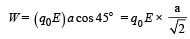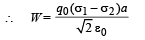Q.23. A conducting liquid bubble of r adius a and th ickn ess t (t << a) is charged to potential V. If the bubble collapses to a droplet, find the potential on the droplet.        (2005 - 2  Marks)

Ans.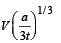Solution. LIQUID BUBBLE : The potential of the liquid bubble is V.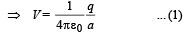where q is the charge on the liquid bubble.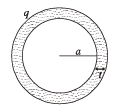LIQUID DROPLET

The volume of liquid droplet = Volume (of the liquid)   in liquid bubble.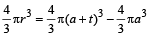or, r3 = a3 + t3 + 3a2t + 3at2 – a3

or, r3 = 3a2t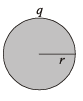(Q t is very small as compared to a)

or, r = [3a2t]1/3 ... (iii)

NOTE : By charge conservation we can conclude that charge on liquid bubble is equal to charge on liquid droplet Let charge on liquid droplet is q.

∴ Potential on liquid droplet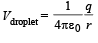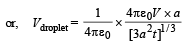[From (i) and (ii)]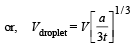The document Subjective Type Questions: Electrostatics- 2 | JEE Advanced Notes | Study Physics 35 Years JEE Main & Advanced Past year Papers - JEE is a part of the JEE Course Physics 35 Years JEE Main & Advanced Past year Papers.
All you need of JEE at this link: JEEUse Code STAYHOME200 and get INR 200 additional OFF Use Coupon Code

Top Courses for JEEPhysics 35 Years JEE Main & Advanced Past year Papers

88 docs|49 tests

Top Courses for JEETrack your progress, build streaks, highlight & save important lessons and more!

,

,

,

,

,

,

,

,

,

,

,

,

,

,

,

,

,

,

,

,

,

;